Algebra
Area
Perimeter
Math Puzzles
Types of Numbers

100

3a = 36. The value of a.

What is 12?

100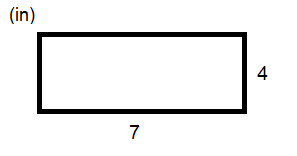The area of this rectangle.

28 in2.

100The perimeter of this rectangle.

What is 20 in?

100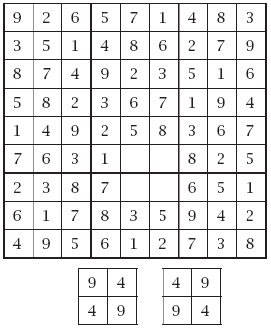Which one fits?

Both of them!!!!!!

100

Any number that can only be multiplied by one and itself.

What are prime numbers?

200

22+b= 12 What is the value of b?

What is 8?

200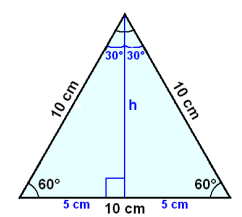The area of this triangle.

What is 50 cm2?

200

If the length of a rectangle is 8 cm and the width is 4 cm, what is the perimeter?

What is 24 cm?

200

Another term for whole numbers.

What are integers?

300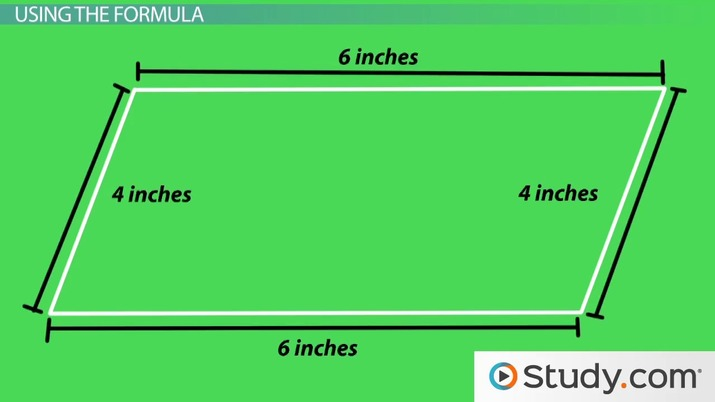The area of this parrelelogram.

24 in2.

300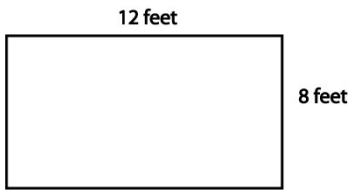The perimeter of this rectangle.

What is 40 feet?

300

A puzzle in which missing numbers are to be filled into a 9 by 9 grid of squares which are subdivided into 3 by 3 boxes so that every row, every column, and every box contains the numbers 1 through 9.

- Merriam Webster

What is sudoku?

300

The famous number that goes on and on.

What is pi?

400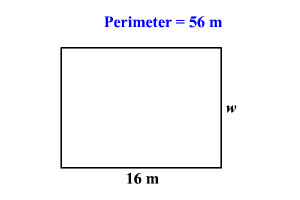The missing width.

What is 10 m?

400

A brand name for a numerical logic puzzle printed on a grid subdivided into clusters of squares, or cages, the object of which is to fill in the squares so that each column and row do not repeat digits, and all the numbers within a cagecombine together using the specified arithmetic operation to equal a target number.

What is Ken ken?

500

a2+b2=c2  Who thought of this theory?

Who is the Pythagoras?

500The area of this cube.

What is 30 in2?

500

This pattern adds the two numbers before it, starting with zero.

What is the Fibonacci Sequence?